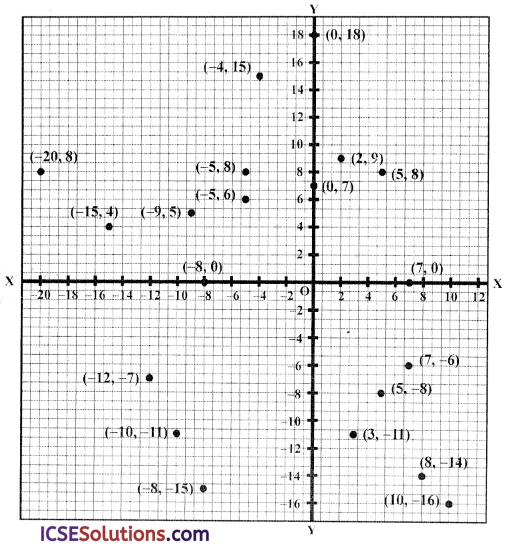Well-structured ICSE S Chand Maths Class 9 Solutions Chapter 20 Coordinates and Graphs of Simultaneous Linear Equations Ex 20(A) facilitate a deeper understanding of mathematical principles.

## S Chand Class 9 ICSE Maths Solutions Chapter 20 Coordinates and Graphs of Simultaneous Linear Equations Ex 20(A)

Question 1.
Plot the following points on a graph paper using the same pair of axes and the same scale for each one.
(i) (0,18)
(ii) (2, 9)
(iii) (5, 8)
(iv) (7, 0)
(v) (5, -8)
(vi) (10,-16)
(Vii) (8, -14)
(viii) (3,-11)
(ix) (-5, 6)
(x) (-15, 4)
(xi) (-20, 8)
(xii) (-9, 5)
(xiii) (-5, 8)
(xiv) (0, 7)
(xv) (-8, -15)
(xvi) (-12, -7)
(xvii) (-10, -11)
(xviii) (-8, 0)
(xix) (-4, 15)
(XX) (7, -6)
Solution:
The points given, have been plotted on the graph given below :Question 2.
The coordinates of the three vertices of a rectangle are given. By plotting the given points, find in each case, the co-ordinates of the fourth vertex.
(i) A (4, 2), B (-2, 2) and D (4, -2);
(ii) B (10, 4), C (0, 4) and D (0, -2).
Solution:
(i) The points A (4, 2), B (-2, 2) and D (4, -2) have been plotted. To complete the rectangle, the point C will be its fourth vertex whose co-ordinates are (-2, -2).(ii) The point B (10, 4), C (0, 4) and D (0, -2) have been plotted on the graph. To complete the rectangle the point A will be its fourth point whose co-ordinates are (10, -2).Question 3.
A (-2, 4), C (4, 10) and D (-2, 10) are the vertices of a square ABCD. Use graphical method to find the coordinates of the fourth vertex B. Also, find
(i) the coordinates of the mid-point of BC,
(ii) the coordinates of the mid-point of CD and
(iii) the coordinates of the point of intersection of the diagonals of the square ABCD.
Solution:
Plot the points A (-2, 4), C (4, 10) and D (-2, 10) on the graph and join them and complete the square
(i) The fourth point is B whose vertices are (4, 4).
(ii) The mid-point of BC will be P whose cocordinates will be (4, 7).
(iii) The mid-point of CD will be Q whose cocordinates will be (1, 10).
(iv) Its diagonals AC and BD intersect each other at O whose co-ordinates will be (1, 7).